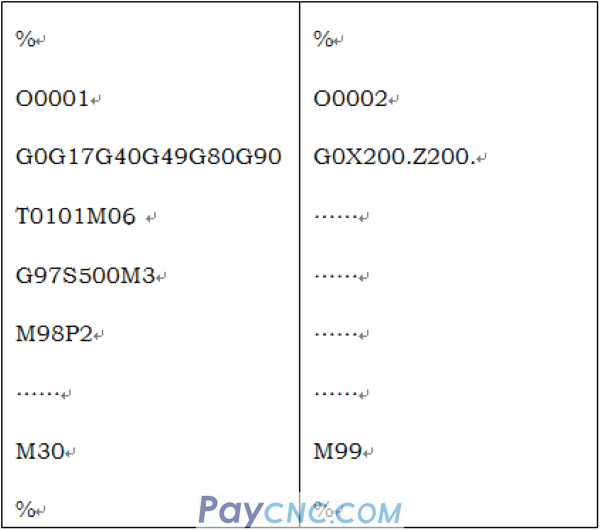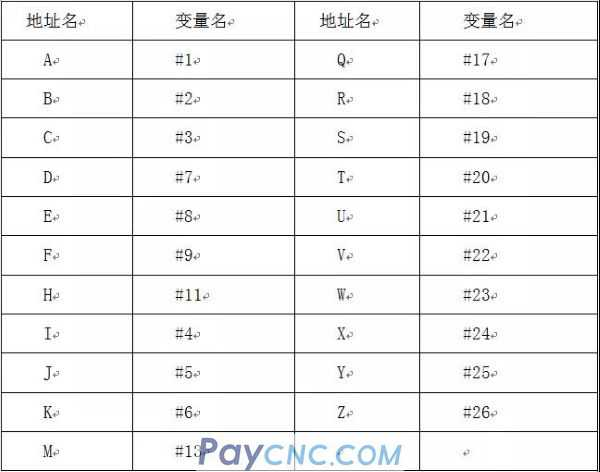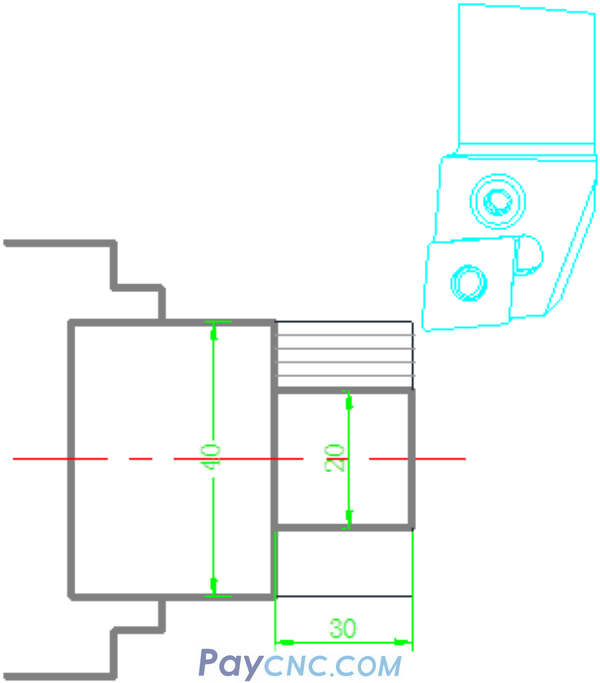The calling of CNC program usually calls subroutines in M98, G65 and other methods.   1. Use M98 to call subroutine   For example, the following two program examples:Among them, O0001 is the main program, and O0002 is the subprogram.   The running sequence of the program is: After running the O0001 program, when running to the M98P2 program segment, the machine tool will jump to the O0002 program   From the beginning of the O0002 program, run the program sequentially from top to bottom, to M99, jump to the main program O0001 to run the block after M98P2.   Two calling methods of the program: Format 1: M98P_ _ _ _ L_   No more than four digits after P_ is the program number;   For example, M98P1, M98P0002, M98P2000L2 L_ is the number of repeated calls (when omitted, it is called 1 time)   Format 2: M98P_ _ _ _ _ _ _   P_ is followed by up to 8 digits, if the following number does not exceed 4 digits, it means that the program is called once   For example, M98P2322 calls O2322 program once.   If the number after P exceeds 4 digits, the last 4 digits are the name of the calling program, and the rest are the number of calls.   For example, M98P00052322 calls the O2322 program 5 times.     Second, use G65 to call a subroutine   G65 belongs to the category of macro program call     Format: G65P subroutine name address name   First look at P. In fact, G65 and M98 subroutine calls are very similar. P is followed by the name of the subroutine.   For example, there is a subroutine O1234, then when the main program is called with G65, it can be written as G65 P1234 (the capital letter O is not needed)   Now it’s time to talk about the very critical address names.   Variables in the macro program are divided into three categories (local variables, public variables, system variables)   Among them, #1～#26 are local variables, and the corresponding relationship between local variables and address names A, B, C...Z is as follows.To put it plainly, the address name is to pass data to its corresponding variable.   For example, the letter A in the above table corresponds to #1, if A10, it is equivalent to passing the data of 10 to variable #1, at this time #1=10;   Similarly, if B20, then #2=20, if Z-26, then #26=-26.   This is a bit vague, I will give you a simple example to give you some inspiration, such as the following parts:For the outer circle of the rough car, I randomly set three local variables #7, #24, #26 to represent:   #7 represents the size of the blank; #24 represents the processed size; #26 represents the length of processing;   The outer circle program for rough turning is as follows: (similar to G90 cycle command) % O0001 G0X#7Z2 WHILE[#7GT#24]DO1 #7=#7-2 G1X#7F#9 Z#26 G0U1. Z2. END1 G91G28Z0 M99 %   Take the above O001 program as a subprogram, and use G65 to call the subprogram as follows:   % O0002 T0101 M13S800 G65P1 D40. X20. Z-30.F0.05 M30 %   When I use G65 to call the program No. O0001, the data after the letters D, X and Z are passed to the corresponding variables.   at this time: D represents the diameter of the part blank;   X represents the size of processing;   Z represents the length of processing;   If processing a similar shape part, we only need to write a main program to call a subroutine.   For example, if the blank is 30, it is processed to D20, and the processing length is -50, the procedure is as follows: % O0002 T0202 M3S1000 G65P1 D30. X20. Z-50.F0.1 M30 %